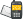It is currently 13 Aug 2020, 17:32### GMAT Club Daily Prep

#### Thank you for using the timer - this advanced tool can estimate your performance and suggest more practice questions. We have subscribed you to Daily Prep Questions via email.

Customized
for You

we will pick new questions that match your level based on your Timer History

Track

every week, we’ll send you an estimated GMAT score based on your performance

Practice
Pays

we will pick new questions that match your level based on your Timer History

#### Not interested in getting valuable practice questions and articles delivered to your email? No problem, unsubscribe here.# GRE Math Challenge #112-If n = pqr, where p, q, and rQuestion banks Downloads My Bookmarks Reviews Important topics
Author Message
TAGS:
Retired ModeratorJoined: 07 Jun 2014
Posts: 4804
GRE 1: Q167 V156WE: Business Development (Energy and Utilities)
Followers: 169

Kudos [?]: 2833 , given: 394

GRE Math Challenge #112-If n = pqr, where p, q, and r [#permalink]
Expert's post00:00

Question Stats:58% (00:55) correct41% (00:15) wrongbased on 17 sessions
If n = pqr, where p, q, and r are three different positive prime numbers, how many different positive divisors does n have, including l and n?

(A) 3
(B) 5
(C) 6
(D) 7
(E) 8
[Reveal] Spoiler: OA

_________________

Sandy
If you found this post useful, please let me know by pressing the Kudos Button

Try our free Online GRE TestInternJoined: 22 Aug 2015
Posts: 6
Followers: 0

Kudos [?]: 5  , given: 0

Re: GRE Math Challenge #112 [#permalink]
1
KUDOS
1 and n makes it 2 divisors
p,q,r makes it another 3
n combinations of 2 from pqr - pq,qr,pr (gives it another 3)

Posted from my mobile deviceInternJoined: 06 Jul 2020
Posts: 8
Followers: 0

Kudos [?]: 1 , given: 1

Re: GRE Math Challenge #112-If n = pqr, where p, q, and r [#permalink]
Another method of solving:

Total # of positive divisors of x^a * y^b * z^c, where x, y, and z are all prime is equal to (a+1) * (b+1) * (c+1)

So if n = p*q*r, and p, q, and r are all prime, then you can also write p*q*r as p^1 * q^1 * r^1.
Therefore, the total # of positive divisors of n = (1+1) * (1+1) * (1+1) = 8

GRE InstructorJoined: 10 Apr 2015
Posts: 3675
Followers: 142

Kudos [?]: 4246 , given: 67

Re: GRE Math Challenge #112-If n = pqr, where p, q, and r [#permalink]
Expert's post
sandy wrote:
If n = pqr, where p, q, and r are three different positive prime numbers, how many different positive divisors does n have, including l and n?

(A) 3
(B) 5
(C) 6
(D) 7
(E) 8

Another approach is to test some values that satisfy the given conditions

n = pqr, where p, q, and r are three different positive prime numbers
Let p = 2
Let q = 3
Let r = 5

So, n = pqr = (2)(3)(5) = 30

How many different positive divisors does n have, including 1 and n?
In other words, "How many different positive divisors does 30 have, including 1 and 30?"

The positive divisors of 30 are: 1, 2, 3, 5, 6, 10, 15 and 30
There are 8 positive divisors

Cheers,
Brent
_________________

Brent Hanneson – Creator of greenlighttestprep.com
If you enjoy my solutions, you'll like my GRE prep course.Re: GRE Math Challenge #112-If n = pqr, where p, q, and r   [#permalink] 29 Jul 2020, 06:13
Display posts from previous: Sort by

# GRE Math Challenge #112-If n = pqr, where p, q, and rQuestion banks Downloads My Bookmarks Reviews Important topicsPowered by phpBB © phpBB Group Kindly note that the GRE® test is a registered trademark of the Educational Testing Service®, and this site has neither been reviewed nor endorsed by ETS®.